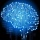Home
IT Knowledge
Inspiration
Languages
EN

# JavaScript - random int in range

4 points
Created by:Root-ssh
121330

Hi, today I would like to show you how to generate random integer number in given range in JavaScript.

## Random int between 0 and 2

``````// ONLINE-RUNNER:browser;

/*
inclusive min (result can be equal to min value)
inclusive max (result can be equal to max value)
*/
function randomizeInteger(min, max) {
return min + Math.floor((max - min + 1) * Math.random());
}

// Example:
console.log(randomizeInteger(0, 2)); // 0
console.log(randomizeInteger(0, 2)); // 2
console.log(randomizeInteger(0, 2)); // 1
console.log(randomizeInteger(0, 2)); // 1``````

If we want to change the range, we just need to call this method with our desired min and max.

About min and max value as stated in a comment:

• inclusive min (result can be equal to min value)
• inclusive max (result can be equal to min value)